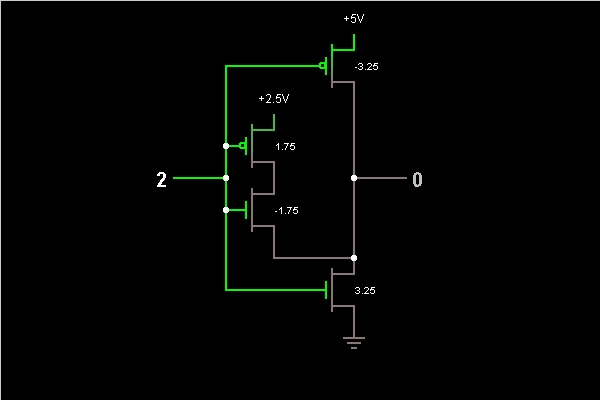# Circuit Simulator - Ternary Logic Inverter

## Where can I get Ternary Logic Inverter Circuit Diagram with Explanation?

IndiaBIX provides you lots of fully solved Ternary Logic Inverter circuit diagram with detailed explanation and working principles.

## How to design a Ternary Logic Inverter (electronic circuit)?

You can easily design the Ternary Logic Inverter circuit by practicing the exercises given below. Here you can design and simulate your own electronic circuits with this Online Circuit Designer and Simulator.### Circuit Description:

This is an example of a ternary logic gate, implemented using MOSFETs that have the indicated threshold voltages. This gate's input has three states: 0 (ground), 1 (2.5 V), and 2 (5 V). This is an inverter; if the input is X, the output is 2-X.

When the input is 2, the n-MOSFET at lower right pulls the output to ground (the MOSFET switches on whenever the gate voltage is 3.25 V or above). When the input is 0, this MOSFET switches off, and the p-MOSFET on top pulls the output to 5 V (the MOSFET switches on whenever the gate voltage is below 5 V-3.25 V, or 1.75 V). When the input is 1, both the MOSFETs on the right are off, but the two MOSFETs on the left switch on, pulling the output to 2.5 V. -- Credits: Mr. Paul Falstad.